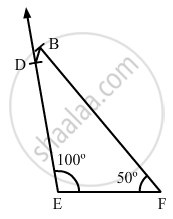Share

# Construct a Quadrilateral Bdef, Where De = 4.5 Cm, Ef = 3.5 Cm, Fb = 6.5 Cm, ∠F = 50° and ∠E = 100°. - Mathematics

Course
ConceptConstructing a Quadrilateral When Two Adjacent Sides and Three Angles Are Known

#### Question

Construct a quadrilateral BDEF, where DE = 4.5 cm, EF = 3.5 cm, FB = 6.5 cm, ∠F = 50° and ∠E = 100°.

#### Solution

Steps of construction:

Step I: Draw EF = 3 . 5 cm .

Step II: Construct ∠DEF = 100° at E .

Step III : With E as the centre and radius 4 . 5 cm, cut off DE = 4 . 5 cm .

Step IV: Construct ∠EFB = 50° at F .

Step V : With F as the centre and radius 6 . 5 cm, cut off FB = 6 . 5 cm .

Step VI: Join BD .